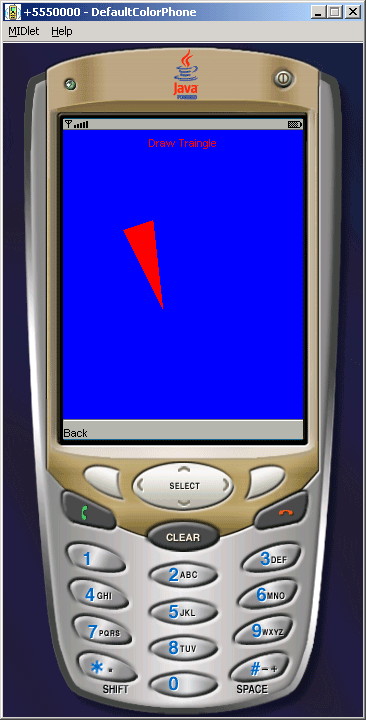Tutorials

# J2ME Draw Triangle, Rectangle, Arc, Line Round Rectangle Example

In this serious of J2ME tutorial, we are going to describe how to draw a triangle, rectangle, arc, line or a round rectangle in one small application.

In this serious of J2ME tutorial, we are going to describe how to draw a triangle, rectangle, arc, line or a round rectangle in one small application.

# J2ME Draw Triangle, Rectangle, Arc, Line Round Rectangle Example

In this serious of J2ME tutorial, we are going to describe how to draw a triangle, rectangle, arc, line or a round rectangle in one small application. Although we have already explained you all these examples separately but in this section we are going to merge them in one. For that we have created a list of all the items that will be used to select the particular item to run. So here we are creating a implicit type list called CanvasDrawList and defined all the elements into it.

Now we need to use the canvas class to draw all the graphic or image. We can call the canvas class in our application through this syntax..

``` class CanvasDraw extends Canvas implements CommandListener```

In this syntax you can see that we are creating a class called CanvasDraw which extends the canvas class through "extend" keyword.

The output of the application will look like as follow....Source Code of CanvasDrawExample.java

 ``` import javax.microedition.midlet.*; import javax.microedition.lcdui.*; public class CanvasDrawExample extends MIDlet implements CommandListener{   private Display display;   private List list;   private Command ok, exit;   private CanvasDraw canvasDraw;   int listItemIndex;   public CanvasDrawExample(){   canvasDraw = new CanvasDraw();   display = Display.getDisplay(this);   list = new List("CanvasDrawList", List.IMPLICIT);   ok = new Command("Draw", Command.OK, 2);   exit = new Command("Exit", Command.EXIT, 2);   list.append("Draw Lines", null);   list.append("Draw Rectangle", null);   list.append("Draw Rounded Rectangle", null);   list.append("Draw Arc", null);   list.append("Draw Triangle", null);   list.addCommand(ok);   list.addCommand(exit);   list.setCommandListener(this);   }      public void startApp(){   display.setCurrent(list);   }   public void pauseApp(){}      public void destroyApp(boolean unconditional){   notifyDestroyed ();   }   public void commandAction(Command c, Displayable d){   listItemIndex = list.getSelectedIndex();   if(c == ok){   display.setCurrent(canvasDraw);   }else if(c == exit){   destroyApp(true);   }   }   class CanvasDraw extends Canvas implements CommandListener{   Command back;   public CanvasDraw(){   back = new Command("Back", Command.BACK, 1);   addCommand(back);   setCommandListener(this);   }   public void paint(Graphics g){   g.setColor(0, 0, 255);   g.fillRect(0,0, getWidth (), getHeight ());   g.setColor(255, 0, 0);   if(listItemIndex == 0){   g.drawString("Draw line", getWidth () / 2, 5, Graphics.HCENTER |  ```  ``` Graphics.TOP);``` ```   g.drawLine(getWidth()/4, 100, 3 * getWidth()/4, 100);   }   if(listItemIndex == 1){   g.drawString("Draw Rectangle", getWidth()/2, 5, Graphics.HCENTER |    Graphics.TOP);``` ```   g.fillRect(getWidth () / 4, 100, getWidth() / 2, 40);   }   if(listItemIndex==2){   g.drawString("Draw Rounded Rectangle", getWidth () / 2, 5,    Graphics.HCENTER | Graphics.TOP);``` ```   g.fillRoundRect(getWidth () / 4, 100, getWidth () / 2, 40, 30, 40);   }   if(listItemIndex == 3){   g.drawString("Draw Arc", getWidth () / 2, 5, Graphics.HCENTER |    Graphics.TOP);  ``` ```   g.fillArc(getWidth () / 4, 100, 90, 90, 180, 270);   }   if(listItemIndex == 4){   g.drawString("Draw Traingle", getWidth () / 2, 5, Graphics.HCENTER |    Graphics.TOP);``` ```   g.fillTriangle(getWidth() / 4, 100, 90, 90, 100, 180);   }   }   public void commandAction (Command c, Displayable d){   if(c == back){   display.setCurrent(list);   }   }   } }```